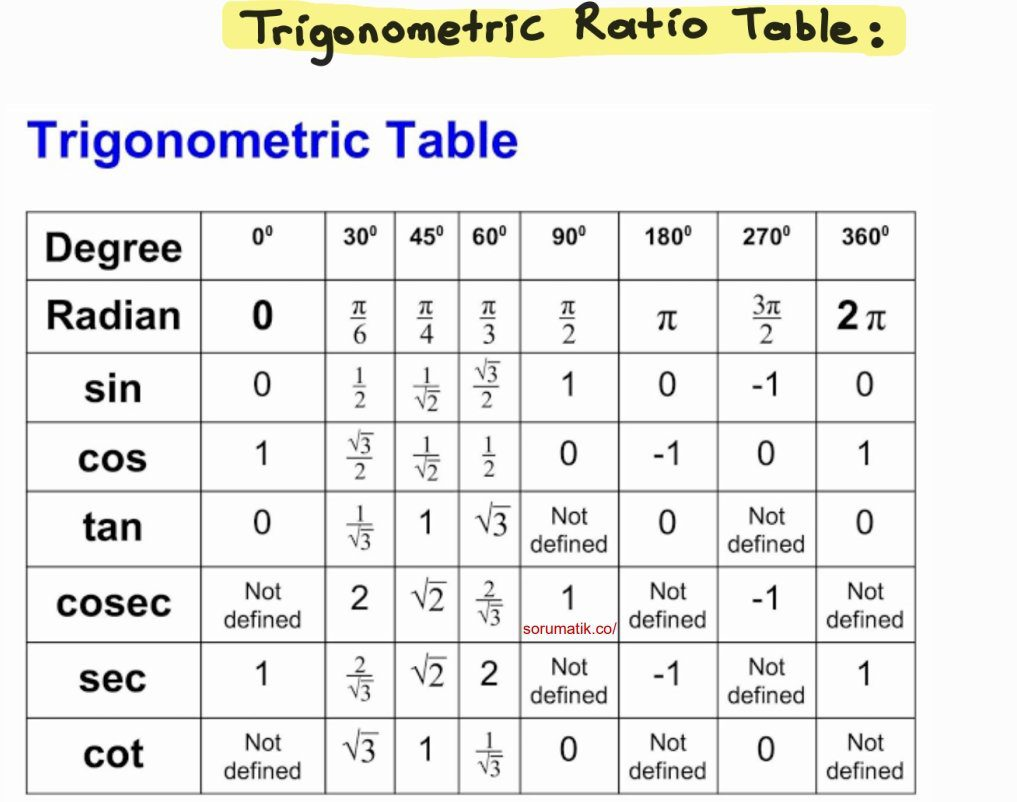math formulas

# List of Trigonometry Formulas PDF

Ücretsiz! Soru Cevap Uygulamamızı kullandın mı? Ziyaret Etmek için Hemen ---> Tıklayınız

Trigonometry formulas are a set of different formulas containing trigonometric identities used to solve problems based on the sides and angles of a right-angled triangle. In our article, we will list the trigonometry formulas. We will see trigonometric identities. You will also be able to download these formulas in PDF form.

İçindekiler

## Basic Trigonometry Formulas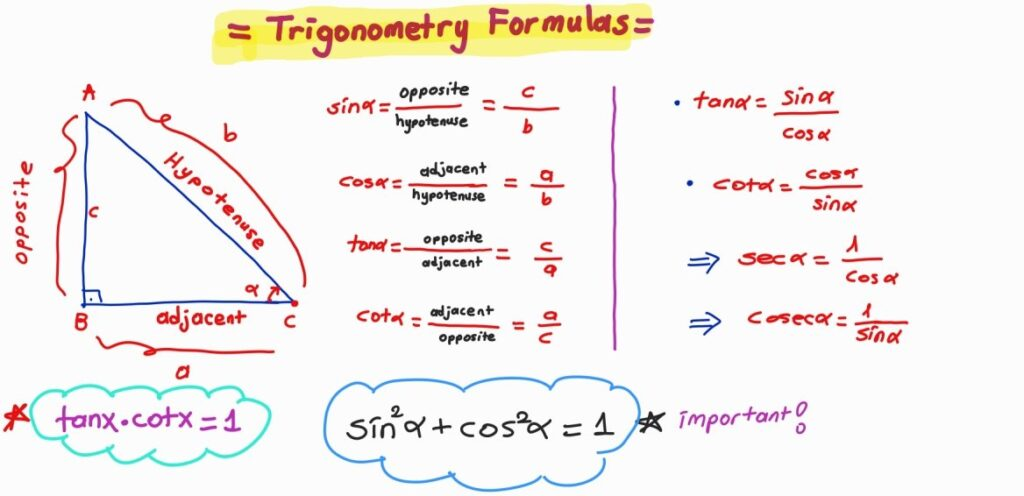#### Trigonometric Ratio Formulas

• sin θ = Perpendicular/Hypotenuse
• cos θ = Base/Hypotenuse
• tan θ = Perpendicular/Base
• sec θ = Hypotenuse/Base
• cosec θ = Hypotenuse/Perpendicular
• cot θ = Base/Perpendicular

### Trigonometry Formulas Involving Reciprocal Identities

• cosec θ = 1/sin θ
• sec θ = 1/cos θ
• cot θ = 1/tan θ
• sin θ = 1/cosec θ
• cos θ = 1/sec θ
• tan θ = 1/cot θ

### Signs of Trigonometric Functions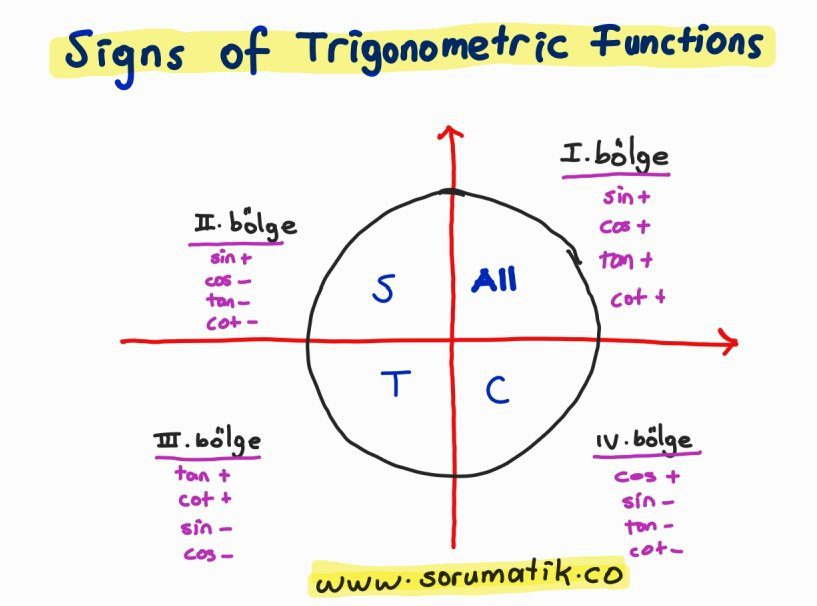### The Law of Sines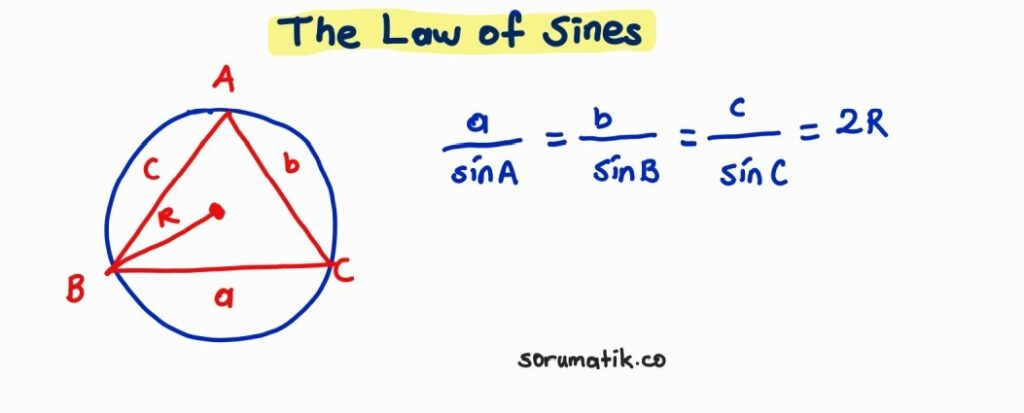### Sine Area Formula

(sin A)/a = (sin B)/b = (sin C)/c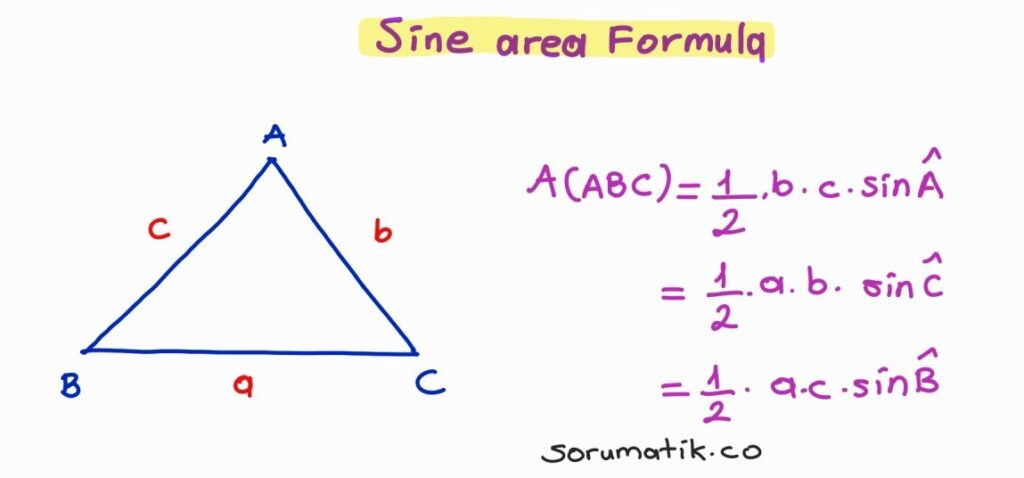Reklam

### The Law of Cosines

• a= b+ c– 2bc cosA
• b= a+ c– 2ac cosB
• c= a+ b 2ab cosC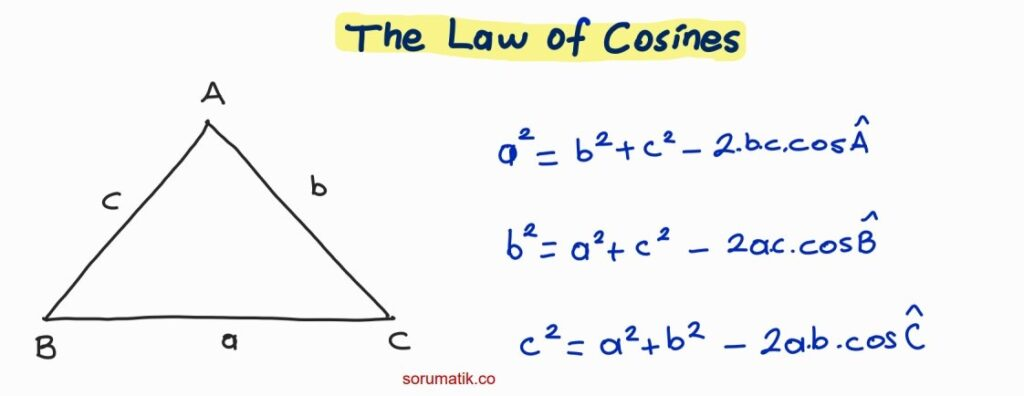### Trigonometry Formulas Involving Sum and Difference Identities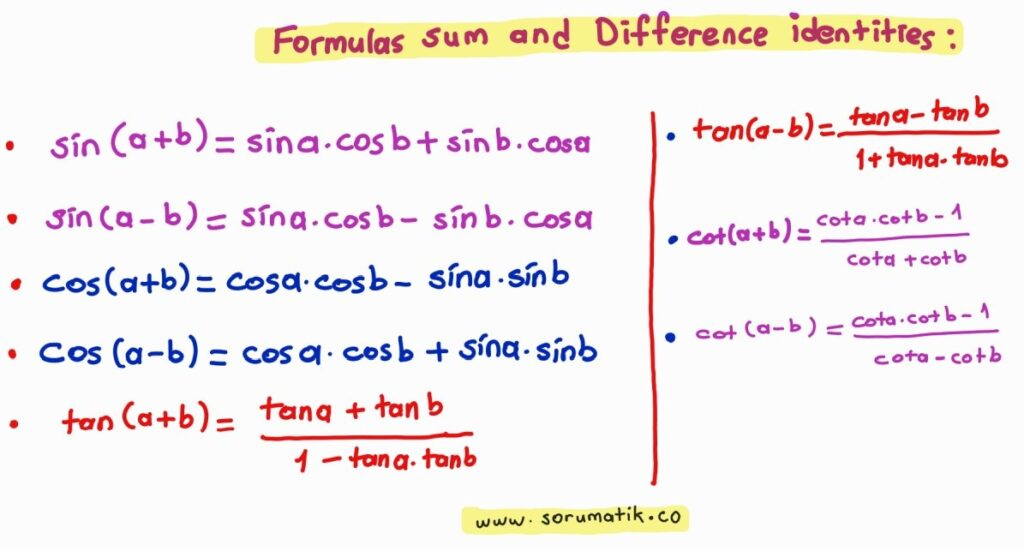### Trigonometry Formulas Involving Double Angle Identities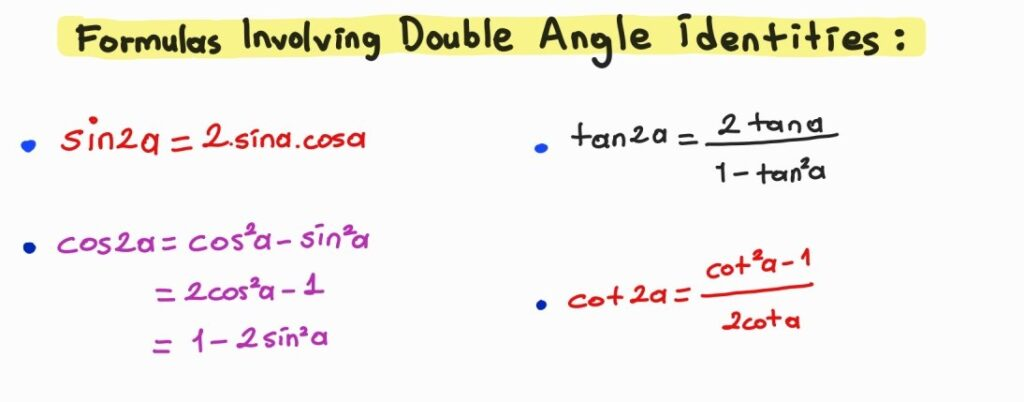### Trigonometry Formulas Involving Half-Angle Identities

sin (x/2) = ±√[(1 – cos x)/2]

cos (x/2) = ± √[(1 + cos x)/2]

tan (x/2) = ±√[(1 – cos x)/(1 + cos x)]

or, tan (x/2) = ±√[(1 – cos x)(1 – cos x)/(1 + cos x)(1 – cos x)]

tan (x/2) = ±√[(1 – cos x)2/(1 – cos2x)]

⇒ tan (x/2) = (1 – cos x)/sin x

### Trigonometry Formulas Involving Sum to Product Identities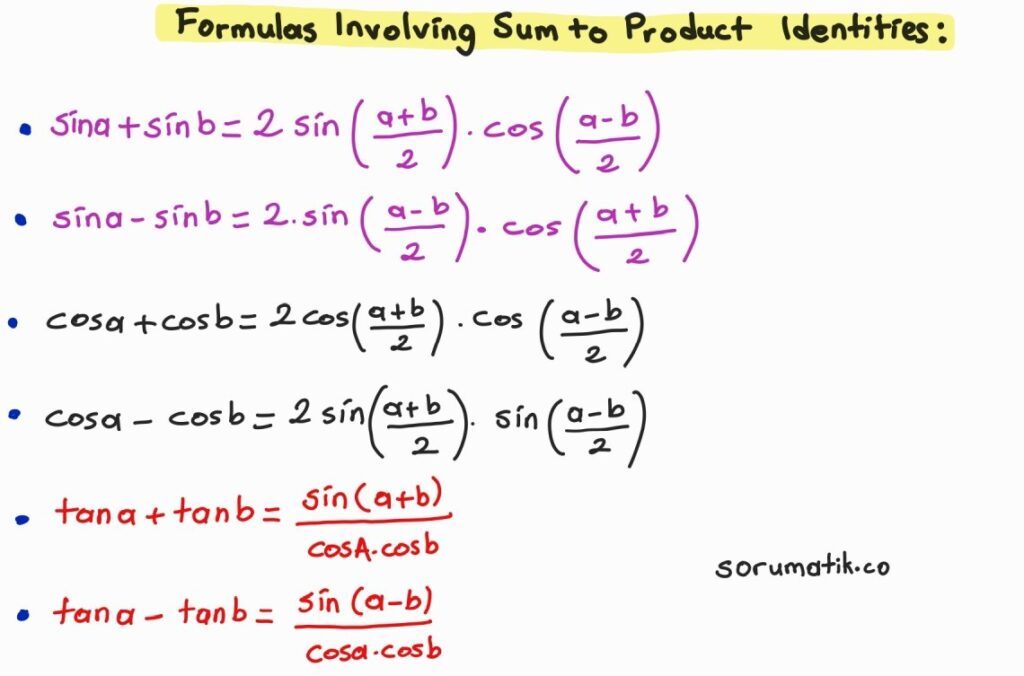### Trigonometry Formulas Involving Product Identities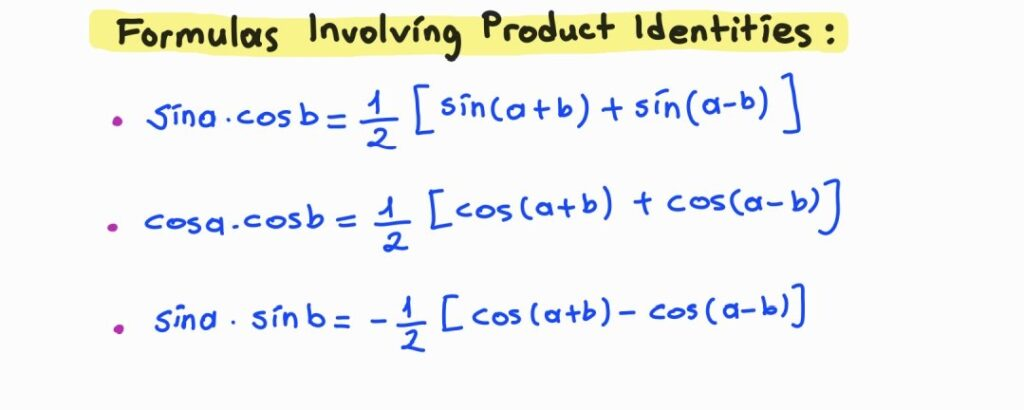### Trigonometry Formulas Involving Periodic Identities(in Radians)

• sin (π/2 – θ) = cos θ
• cos (π/2 – θ) = sin θ
• sin (π/2 + θ) = cos θ
• cos (π/2 + θ) = – sin θ

• sin (3π/2 – θ) = – cos θ
• cos (3π/2 – θ) = – sin θ
• sin (3π/2 + θ) = – cos θ
• cos (3π/2 + θ) = sin θ

• sin (π – θ) = sin θ
• cos (π – θ) = – cos θ
• sin (π + θ) = – sin θ
• cos (π + θ) = – cos θ

• sin (2π – θ) = – sin θ
• cos (2π – θ) = cos θ
• sin (2π + θ) = sin θ
• cos (2π + θ) = cos θ

### Trigonometry Formulas Involving Co-function Identities(in Degrees)

• sin(90° − x) = cos x
• cos(90° − x) = sin x
• tan(90° − x) = cot x
• cot(90° − x) = tan x
• sec(90° − x) = cosec x
• cosec(90° − x) = sec x

### Trigonometry Formulas Involving Reciprocal Identities

• cosec θ = 1/sin θ
• sec θ = 1/cos θ
• cot θ = 1/tan θ
• sin θ = 1/cosec θ
• cos θ = 1/sec θ
• tan θ = 1/cot θ

### Inverse Trigonometry Formulas

• sin-1 (-x) = -sin-1 x
• cos-1 (-x) = π – cos-1 x
• tan-1 (-x) = -tan-1 x
• cosec-1 (-x) = -cosec-1 x
• sec-1 (-x) = π – sec-1 x
• cot-1 (-x) = π – cot-1 x

### Trigonometry Formulas Involving Triple Angle Identities

• sin 3x = 3sin x – 4sin3x
• cos 3x = 4cos3x – 3cos x
• tan 3x = [3tanx – tan3x]/[1 – 3tan2x]

## Trigonometric Ratio Table